# ₦airaland Forum

Welcome, Guest: Join Nairaland / LOGIN! / Trending / Recent / New
Stats: 2,210,238 members, 4,823,990 topics. Date: Wednesday, 20 March 2019 at 06:11 AM

## What Is The Total Weights Of The Animals In This Picture? - Education (2) - Nairaland

(1) (2) (3) (4) (Reply) (Go Down)

 Re: What Is The Total Weights Of The Animals In This Picture? by ShakurM(m): 1:32pm On Feb 13, 2015 The total weight of all the animals in the picture is twenty seven (27) grams or 27,000 kilograms or 59.5 pounds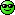Re: What Is The Total Weights Of The Animals In This Picture? by Posman(m): 1:32pm On Feb 13, 2015 this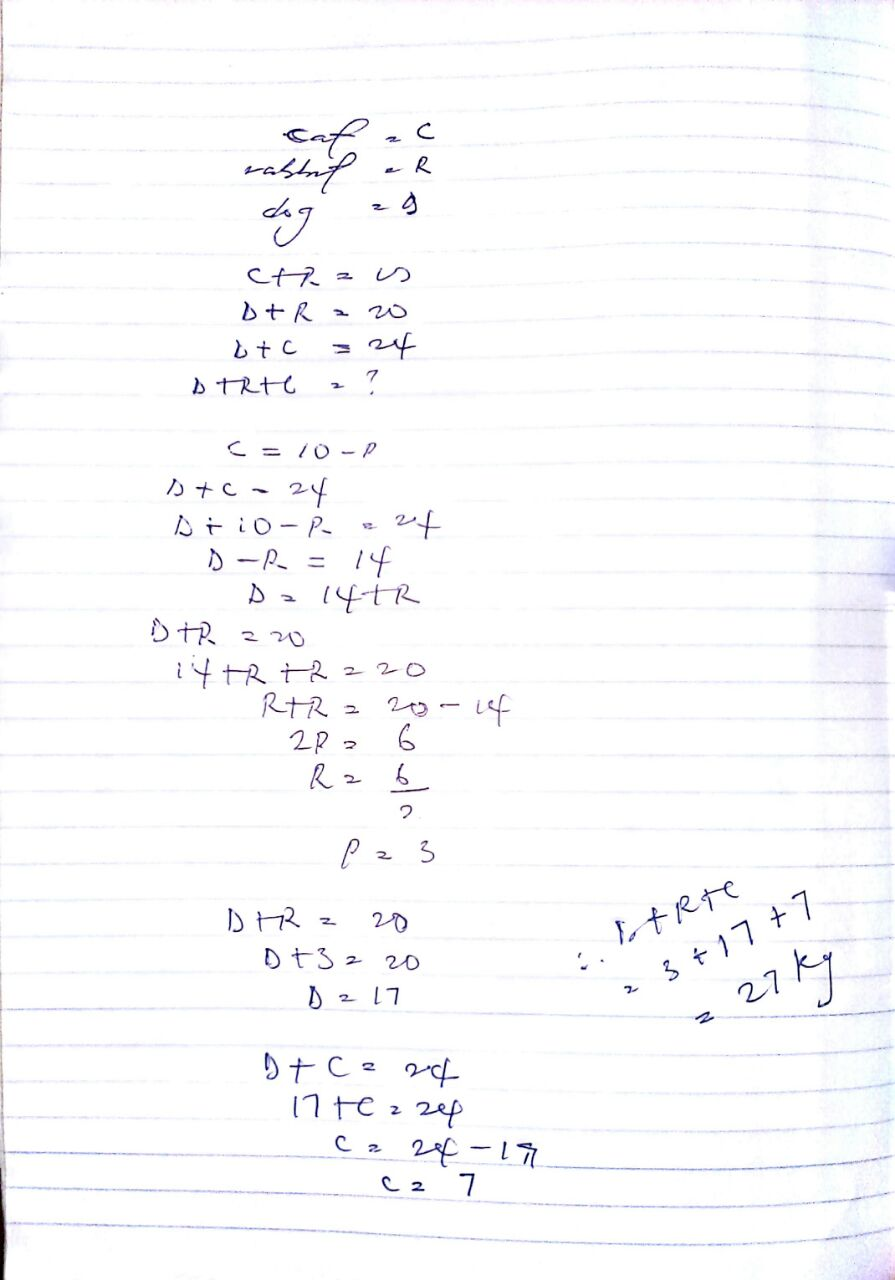Re: What Is The Total Weights Of The Animals In This Picture? by M4gunners: 1:32pm On Feb 13, 2015 Modified fast fast give me some mints to crack brain again e don tail Re: What Is The Total Weights Of The Animals In This Picture? by mployer(m): 1:33pm On Feb 13, 2015 27kg.Simultaneous equation in three variables. Re: What Is The Total Weights Of The Animals In This Picture? by siegfried99(m): 1:33pm On Feb 13, 2015 **brb** Re: What Is The Total Weights Of The Animals In This Picture? by franciskaine(m): 1:33pm On Feb 13, 2015 As I no sabi maths I use common logic. 27 is the answer, it took me like 3mins sha. Re: What Is The Total Weights Of The Animals In This Picture? by Monkey97: 1:34pm On Feb 13, 2015 if u get mb, Google go sabi d answer Re: What Is The Total Weights Of The Animals In This Picture? by dammyconcept(m): 1:34pm On Feb 13, 2015 oh my God !simple calculation just that I can't solve now because my teacher refused to teach us simple calculation because PDP strategy refuse to pay their salaryuntil Jonathan pay teacher salary I will solve it for u 1 Like 1 Share Re: What Is The Total Weights Of The Animals In This Picture? by RedArrow: 1:34pm On Feb 13, 2015 Dog = 17kgCat = 7kgRabbit = 3kgTotal = 27kg Re: What Is The Total Weights Of The Animals In This Picture? by delman88(m): 1:34pm On Feb 13, 2015 husseinjnr:Let A= Rabbit B= Cat C = dogFrom Figure above, in simple analysis A + B = 10kg -----------equation 1A + C = 20kg -----------equation 2B + C = 24 kg ---------- equation 3From equation 2, A= 20- CFrom equation 3B= 24- CSubstitute A and B into equation 120-C + 24-C =1044-2C = 10-2C= 10-44-2C = -34C= 34/2C= 17Substitute C into equation 2A+ 17 = 20A= 3Substitute C into equation 3B+17 = 24B= 7ThereforeA= 3, B= 7, C= 17Total weight is 3+7+17 = 27Rabbit= 3kgCat = 7kgDog = 17kgwetin naaa>>> we know say you go school!!! 1 Like Re: What Is The Total Weights Of The Animals In This Picture? by Nobody: 1:35pm On Feb 13, 2015 They all look skinny. Wait leme feed them and reweigh.DEAR NAIRALANDERS, DOWNLOAD MY REMIX OF CHRIS BROWN'S "don't Wake Me Up" HERE: https://my.notjustok.com/track/download/id/7578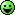Re: What Is The Total Weights Of The Animals In This Picture? by Eluwilussit(m): 1:36pm On Feb 13, 2015 Better than GEJ and GMB matter. Re: What Is The Total Weights Of The Animals In This Picture? by ArchEnemy(m): 1:38pm On Feb 13, 2015 husseinjnr:Let A= Rabbit B= Cat C = dogFrom Figure above, in simple analysis A + B = 10kg -----------equation 1A + C = 20kg -----------equation 2B + C = 24 kg ---------- equation 3From equation 2, A= 20- CFrom equation 3B= 24- CSubstitute A and B into equation 120-C + 24-C =1044-2C = 10-2C= 10-44-2C = -34C= 34/2C= 17Substitute C into equation 2A+ 17 = 20A= 3Substitute C into equation 3B+17 = 24B= 7ThereforeA= 3, B= 7, C= 17Total weight is 3+7+17 = 27Rabbit= 3kgCat = 7kgDog = 17kggive this man a pack of hollandia yoghurt 3 Likes Re: What Is The Total Weights Of The Animals In This Picture? by obitobe: 1:40pm On Feb 13, 2015 this is just a simple linear equation problem, the total weight is 27kg Re: What Is The Total Weights Of The Animals In This Picture? by M4gunners: 1:40pm On Feb 13, 2015 27 i guess not really dat hard Re: What Is The Total Weights Of The Animals In This Picture? by omoadeleye(m): 1:44pm On Feb 13, 2015 Lol, tnj God mai brain no really dull, maje i no stress maiself post d answer again Re: What Is The Total Weights Of The Animals In This Picture? by piagetskinner(m): 1:45pm On Feb 13, 2015 No need for all this long calc...27! Re: What Is The Total Weights Of The Animals In This Picture? by Onozboy(m): 1:45pm On Feb 13, 2015 apoti: 27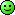show ur working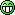Re: What Is The Total Weights Of The Animals In This Picture? by Misogynist2014(m): 1:45pm On Feb 13, 2015 Hysmady:Cat 7Rabbit 3Dog 17 That's how they fail exams. Re: What Is The Total Weights Of The Animals In This Picture? by Alore: 1:46pm On Feb 13, 2015 husseinjnr:Let A= Rabbit B= Cat C = dogFrom Figure above, in simple analysis A + B = 10kg -----------equation 1A + C = 20kg -----------equation 2B + C = 24 kg ---------- equation 3From equation 2, A= 20- CFrom equation 3B= 24- CSubstitute A and B into equation 120-C + 24-C =1044-2C = 10-2C= 10-44-2C = -34C= 34/2C= 17Substitute C into equation 2A+ 17 = 20A= 3Substitute C into equation 3B+17 = 24B= 7ThereforeA= 3, B= 7, C= 17Total weight is 3+7+17 = 27Rabbit= 3kgCat = 7kgDog = 17kgTHIS is the mathematical and most analytical way to answer the question. Good job! 3 Likes 1 Share Re: What Is The Total Weights Of The Animals In This Picture? by Tymax(m): 1:49pm On Feb 13, 2015 Best answer so far. Concise! The rest of you wasted time getting the individual weights.lifestyle1: Lets assume rabbit, cat and dog’s weight are R,C and D correspondingly.then we can sayEquation 1: R + C = 10Equation 2: D + R = 20Equation 3: C + D= 24Then we need to find value of C + R + DOn adding Equation 1,2 and 32R + 2 C + 2D = 54 => R+C+D = 27Na expo i use o1 Like Re: What Is The Total Weights Of The Animals In This Picture? by 9jaADMIN(m): 1:51pm On Feb 13, 2015 Cat = 7kgDog = 17kgRabbit = 3kgif the first pics is CAT and RABBIT : 10kg , then 7kg + 3kg = 10kg Then te 2nd pics is Dog and Rabbit : 20kgThen 17kg + 3kg = 20kg Then the 3rd pics Dog and Cat : 24kgThen 17kg + 7kg = 24kgThe last include all the animals which is Dog , Cat , Rabbit :- 17kg + 7kg + 3kg = 27kg Thanks for this stuff Re: What Is The Total Weights Of The Animals In This Picture? by ebiagforum(m): 1:52pm On Feb 13, 2015 dammyconcept:oh my God !simple calculation just that I can't solve now because my teacher refused to teach us simple calculation because PDP strategy refuse to pay their salaryuntil Jonathan pay teacher salary I will solve it for u Don't worry, GMB is coming to pay teacher's salary. Vote4Change Re: What Is The Total Weights Of The Animals In This Picture? by Realdeals(m): 1:53pm On Feb 13, 2015 husseinjnr:Let A= Rabbit B= Cat C = dogFrom Figure above, in simple analysis A + B = 10kg -----------equation 1A + C = 20kg -----------equation 2B + C = 24 kg ---------- equation 3From equation 2, A= 20- CFrom equation 3B= 24- CSubstitute A and B into equation 120-C + 24-C =1044-2C = 10-2C= 10-44-2C = -34C= 34/2C= 17Substitute C into equation 2A+ 17 = 20A= 3Substitute C into equation 3B+17 = 24B= 7ThereforeA= 3, B= 7, C= 17Total weight is 3+7+17 = 27Rabbit= 3kgCat = 7kgDog = 17kgEFIWE!Like we use to in my secondary school days Re: What Is The Total Weights Of The Animals In This Picture? by funnyme2015(f): 1:53pm On Feb 13, 2015 Hysmady: You're funny...BtW I love ur package @ ur DP Re: What Is The Total Weights Of The Animals In This Picture? by boss096(m): 1:54pm On Feb 13, 2015 27kg. Bt wot if an animal is selected at random wat is d prob. That d animal is neither c nr r.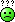Re: What Is The Total Weights Of The Animals In This Picture? by Rotty32: 1:58pm On Feb 13, 2015 Simply 27KG Re: What Is The Total Weights Of The Animals In This Picture? by Fash0622: 1:59pm On Feb 13, 2015 . Re: What Is The Total Weights Of The Animals In This Picture? by dechandel(f): 2:00pm On Feb 13, 2015 kilokeys:lol.. i stumbled on this q last week.. in d dead of d night. went tthrough stress solving it.. while my e-bae dechandel made a good guess using a shorter method.. i almost cried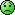When i tell you to apply logical reasoning in life.. I know what i am sayingImagine when a law student beat an engineering graduate in a maths essay(1) (2) (3) (4) (Reply)

(Go Up)

 Sections: politics (1) business autos (1) jobs (1) career education (1) romance computers phones travel sports fashion health religion celebs tv-movies music-radio literature webmasters programming techmarket Nairaland - Copyright © 2005 - 2019 Oluwaseun Osewa. All rights reserved. See How To Advertise. 75Disclaimer: Every Nairaland member is solely responsible for anything that he/she posts or uploads on Nairaland.